# HSSlive: Plus One & Plus Two Notes & Solutions for Kerala State Board

## AP Board Class 7 Maths Chapter 13 Area and Perimeter Ex 6 Textbook Solutions PDF: Download Andhra Pradesh Board STD 7th Maths Chapter 13 Area and Perimeter Ex 6 Book AnswersAP Board Class 7 Maths Chapter 13 Area and Perimeter Ex 6 Textbook Solutions PDF: Download Andhra Pradesh Board STD 7th Maths Chapter 13 Area and Perimeter Ex 6 Book Answers

## Andhra Pradesh State Board Class 7th Maths Chapter 13 Area and Perimeter Ex 6 Books Solutions

 Board AP Board Materials Textbook Solutions/Guide Format DOC/PDF Class 7th Subject Maths Chapters Maths Chapter 13 Area and Perimeter Ex 6 Provider Hsslive

2. Click on the Andhra Pradesh Board Class 7th Maths Chapter 13 Area and Perimeter Ex 6 Answers.
3. Look for your Andhra Pradesh Board STD 7th Maths Chapter 13 Area and Perimeter Ex 6 Textbooks PDF.
4. Now download or read the Andhra Pradesh Board Class 7th Maths Chapter 13 Area and Perimeter Ex 6 Textbook Solutions for PDF Free.

## AP Board Class 7th Maths Chapter 13 Area and Perimeter Ex 6 Textbooks Solutions with Answer PDF Download

Find below the list of all AP Board Class 7th Maths Chapter 13 Area and Perimeter Ex 6 Textbook Solutions for PDF’s for you to download and prepare for the upcoming exams:

Question 1.
A path 2.5 m wide is running around a square field whose side is 45 m. Determine the area of the path.
Solution: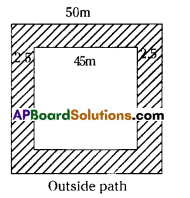Area of the path= (Area of outer figure) – (Area of inner figure)
= (50 × 50) – (45 × 45)
= 2500 – 2025 = 475 m2

Question 2.
The central hail ofa school is 18m long and 12.5 m wide. A carpet is to be laid on the floor leaving a strip 50 cm wide near the walls, uncovered. Find the area of the carpet and also the uncovered portion?
Solution: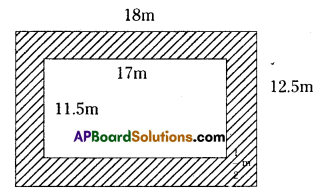Given
Length of the hail = 18m
Width of the strip = 50cm = 12m
Length of inner rectangle = 18 – (12 + 12) 17m
Breadth of inner rectangle = 12.5 – (12 + 12) = 11.5 m
Area of the strip = (Area of the outer fIgure) – (Area of the inner figure)
= 18 × 12.5 – 17 ×11.5
= 225 – 195.5 = 29.5 m2
∴ Area of the carpet = 195.5 m2

Question 3.
The length of the side of a grassy square plot is 80 m. Two walking paths each 4m wide
are constructed parallel to the sides of the plot such that they cut each other at the centre
of the plot. Determine the area of the paths.
Solution: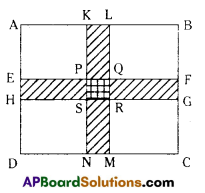Given : A square grassary plot of length 80m
Two paths of width 4m each.
From the question
KL = 4m and KN = 80m
EH = 4m and EF 80m
PQ = 4m and PS 4m
Area of two paths = 🖾 KLMN + 🖾 EFGH – 🖾 FQRS
= KN x KL + EF × EH – PQ × PS
= 80 × 4 + 80 × 4 – 4 × 4
= 320 + 320 – 16
= 624sq.m.

Question 4.
A verandah 2 m wide is constructed all around a room of dimensions 8 m X 5 m. Find the area of the verandah
Solution: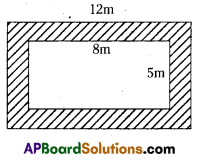Length of outer rectangle = 8 + 2 + 2 =12m
Breadth of outer rectangle = 5 + 2 + 2 = 9m
Area of outer rectangle = l x b = 12 × 9 = 108m2
Length of inner rectangle = 8m
Breadth of inner rectangle = 5m
Area of inner rectangle = l x b = 8 × 5 = 40m2
:. Area of the verandah = (Area of outer figure) – (Area of inner figure)
= 108 – 40 = 68m2

Question 5.
The length of a rectangular park is 700 m and its breadth is 300 m. Two crossroads, each of width 10 m, cut the centre of a rectangular park and are parallel to its sides. Find the area of the roads. Also, find the area of the park excluding the area of the crossroads.
Solution: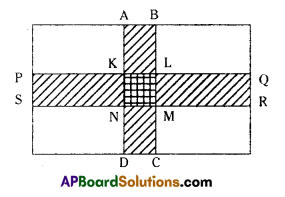Given
Length of the rectangular park = 700 m
Breadth of the rectangular park = 300 ni
From the question,
PS 10m. PQ = 700 ni
Area of 🖾 PQRS = 10 × 700 = 7000m2
AB = 10m, AD = 300m
Area of 🖾 ABCD = 300 x 10 3000m2
KL = 10m, KN = 10m
🖾 KLMN = 10 × 10 = 100m2
Area of the two paths = 🖾 IQRS + 🖾 AF3CD – 🖾 KLMN
= 7000 + 3000 – 100
= 9900m2
The remaining area of the park = Area of the whole park – Area of the two paths
= 700 × 300 – 9900
= 210000 – 9900
= 200100 q.m.

## Andhra Pradesh Board Class 7th Maths Chapter 13 Area and Perimeter Ex 6 Textbooks for Exam Preparations

Andhra Pradesh Board Class 7th Maths Chapter 13 Area and Perimeter Ex 6 Textbook Solutions can be of great help in your Andhra Pradesh Board Class 7th Maths Chapter 13 Area and Perimeter Ex 6 exam preparation. The AP Board STD 7th Maths Chapter 13 Area and Perimeter Ex 6 Textbooks study material, used with the English medium textbooks, can help you complete the entire Class 7th Maths Chapter 13 Area and Perimeter Ex 6 Books State Board syllabus with maximum efficiency.

## FAQs Regarding Andhra Pradesh Board Class 7th Maths Chapter 13 Area and Perimeter Ex 6 Textbook Solutions

#### Can we get a Andhra Pradesh State Board Book PDF for all Classes?

Yes you can get Andhra Pradesh Board Text Book PDF for all classes using the links provided in the above article.

## Important Terms

Andhra Pradesh Board Class 7th Maths Chapter 13 Area and Perimeter Ex 6, AP Board Class 7th Maths Chapter 13 Area and Perimeter Ex 6 Textbooks, Andhra Pradesh State Board Class 7th Maths Chapter 13 Area and Perimeter Ex 6, Andhra Pradesh State Board Class 7th Maths Chapter 13 Area and Perimeter Ex 6 Textbook solutions, AP Board Class 7th Maths Chapter 13 Area and Perimeter Ex 6 Textbooks Solutions, Andhra Pradesh Board STD 7th Maths Chapter 13 Area and Perimeter Ex 6, AP Board STD 7th Maths Chapter 13 Area and Perimeter Ex 6 Textbooks, Andhra Pradesh State Board STD 7th Maths Chapter 13 Area and Perimeter Ex 6, Andhra Pradesh State Board STD 7th Maths Chapter 13 Area and Perimeter Ex 6 Textbook solutions, AP Board STD 7th Maths Chapter 13 Area and Perimeter Ex 6 Textbooks Solutions,
Share: